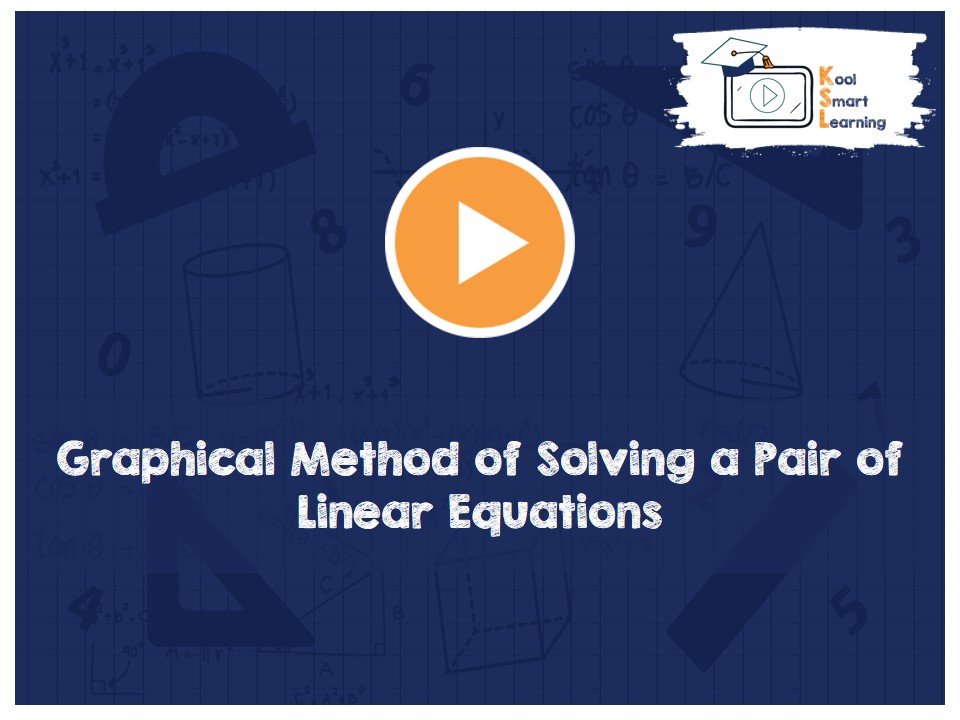At KoolSmartLearning, we intend to harness the power of online education to make learning easy.## Graphical Method of Solving a Pair of Linear Equations

/  Graphical Method of Solving a Pair of Linear Equations## Graphical Method of Solving a Pair of Linear Equations

The video discusses the Geometrical representation of a Pair of Linear Equations in Two Variables & how it can be used to solve the equations. It also talks about different conditions of the pair of linear equations in two variables like lines intersecting at a point, parallel lines & coinciding lines, consistent & inconsistent systems and area bound by the two equations and the axes.

More Related Videos